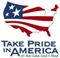Scientific Investigations Report 2008–5025

U.S. GEOLOGICAL SURVEY
Scientific Investigations Report 2008–5025

## Conversion Factors and Datums

### Conversion Factors

Inch/Pound to SI

Multiply By To obtain
inch (in.) 2.54 centimeter (cm)
inch (in.) 25.4 millimeter (mm)
foot (ft) 0.3048 meter (m)
acre 0.004047 square kilometer (km2)
square mile (mi2) 2.590 square kilometer (km2)

SI to Inch/Pound

Multiply By To obtain
meter (m) 3.281 foot (ft)
kilometer (km) 0.6214 mile (mi)
kilometer (km) 0.5400 mile, nautical (nmi)
meter (m) 1.094 yard (yd)
square kilometer (km2) 247.1 acre
square kilometer (km2) .0386 square mile (mi2)

Temperature in degrees Celsius (°C) may be converted to degrees Fahrenheit (°F) as follows:

°F=(1.8×°C)+32.

Temperature in degrees Fahrenheit (°F) may be converted to degrees Celsius (°C) as follows:

°C=(°F-32)/1.8.

Concentrations of chemical constituents in water are given either in milligrams per liter (mg/L) or micrograms per liter (µg/L).

### Datums

Vertical coordinate information is referenced to the North American Vertical Datum of 1988 (NAVD 88).

Horizontal coordinate information is referenced to the North American Datum of 1983 (NAD 83).

Altitude, as used in this report, refers to distance above the vertical datum.U.S. Department of the Interior | U.S. Geological Survey